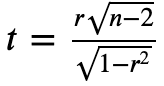# Signficance of mean correlation matrix

I’m following this nilearn tutorial and I was looking for a way to get a significance value for the `mean_correlation_matrix`, where each cell would tell me if the correlation between the ROIs was significantly greater than chance. Is this possible in nilearn?

Could be wrong, but here are some thoughts:

On an individual level, that is, for each connectivity matrix before taking the mean, you can calculate a per-connection t-statistic with the formula. From there you can calculate whether individual connections are significant, after appropriately adjusting your alpha value for multiple comparisons.

Across all participants (e.g. the mean_correlation_matrix), instead of looking at just the mean, you can look at the distribution of r-values that make up each cell. Treating each cell as a different population, you can run a one-sample t-test to test whether the average r-value is different than 0 in a given cell. You might want to limit your matrices to only an upper/lower triangular version to cut redundancies, making multiple comparison correction less stringent.

Steven

Technically, the above answer is correct (although it is actually hard what is the right value for n: it should correspond to the degrees of freedom in the signal after filtering).
However, I would emphasize that this test is again a silly null hypothesis, namely that there is no correlation between brain regions. I would warn again strong interpretation of a “significant” statistic.
HTH,
Bertrand

2 Likes

Here is another approach that has been implemented into the AFNI program MBA:

Gang